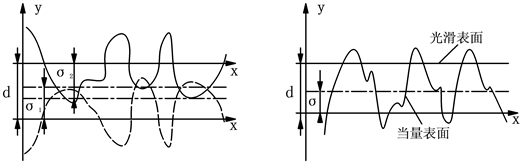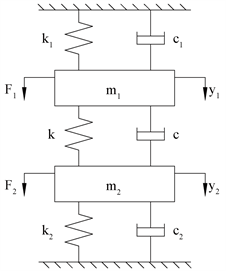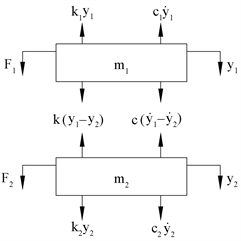﻿ 结合面接触特性检测模型振动响应

# 结合面接触特性检测模型振动响应Vibration Response of Detection Model for Detecting Contact Characteristics of Joint Surface

Abstract: The research of this paper is based on two degree of freedom vibration model, through mathe-matical derivation and experimental research to obtain the law of partial influence of contact stiffness k and contact damping c, so the aim of research is to explore a kind of combination surface contact parameter detection method based on the two degree of freedom vibration system, which will provide the certain theoretical support and experimental basis for the subsequent research in contact feature of combination surface.

1. 引言

2. 结合面检测模型的建立

G-W接触模型(a) 实际接触 (b) 等效模型

Figure 1. Contacts of rough surface

3. 二自由度振动系统物理模型Figure 2. Two degree of freedom vibration system model diagramFigure 3. Two degree of freedom vibration system force analysis chart

$\left\{\begin{array}{l}{F}_{1}-{k}_{1}{y}_{1}-{c}_{1}{\stackrel{˙}{y}}_{1}-k\left({y}_{1}-{y}_{2}\right)-c\left({\stackrel{˙}{y}}_{1}-{\stackrel{˙}{y}}_{2}\right)={m}_{1}{\stackrel{¨}{y}}_{1}\\ {F}_{2}-{k}_{2}{y}_{2}-{c}_{2}{\stackrel{˙}{y}}_{2}-k\left({y}_{1}-{y}_{2}\right)-c\left({\stackrel{˙}{y}}_{1}-{\stackrel{˙}{y}}_{2}\right)={m}_{2}{\stackrel{¨}{y}}_{2}\end{array}$ (2.1)

$\left\{\begin{array}{l}-{k}_{1}{y}_{1}-{c}_{1}{\stackrel{˙}{y}}_{1}-k\left({y}_{1}-{y}_{2}\right)-c\left({\stackrel{˙}{y}}_{1}-{\stackrel{˙}{y}}_{2}\right)={m}_{1}{\stackrel{¨}{y}}_{1}\\ -{k}_{2}{y}_{2}-{c}_{2}{\stackrel{˙}{y}}_{2}-k\left({y}_{1}-{y}_{2}\right)-c\left({\stackrel{˙}{y}}_{1}-{\stackrel{˙}{y}}_{2}\right)={m}_{2}{\stackrel{¨}{y}}_{2}\end{array}$ (2.2)

4. 结合面接触特性检测模型振动响应

$\left\{\begin{array}{l}{m}_{1}{\stackrel{¨}{y}}_{1}-{k}_{1}{y}_{1}-{c}_{1}{\stackrel{˙}{y}}_{1}-k\left({y}_{1}-{y}_{2}\right)-c\left({\stackrel{˙}{y}}_{1}-{\stackrel{˙}{y}}_{2}\right)=0\\ {m}_{2}{\stackrel{¨}{y}}_{2}-{k}_{2}{y}_{2}-{c}_{2}{\stackrel{˙}{y}}_{2}-k\left({y}_{1}-{y}_{2}\right)-c\left({\stackrel{˙}{y}}_{1}-{\stackrel{˙}{y}}_{2}\right)=0\end{array}$ (3.1)

$\left[\begin{array}{cc}{m}_{1}& 0\\ 0& {m}_{2}\end{array}\right]\left\{\begin{array}{c}{\stackrel{¨}{y}}_{1}\\ {\stackrel{¨}{y}}_{2}\end{array}\right\}+\left[\begin{array}{cc}{c}_{1}+c& -c\\ -c& {c}_{2}+c\end{array}\right]\left\{\begin{array}{c}{\stackrel{˙}{y}}_{1}\\ {\stackrel{˙}{y}}_{2}\end{array}\right\}+\left[\begin{array}{cc}{k}_{1}+k& -k\\ -k& {k}_{2}+k\end{array}\right]\left\{\begin{array}{c}{y}_{1}\\ {y}_{2}\end{array}\right\}=\left\{\begin{array}{c}0\\ 0\end{array}\right\}$ (3.2)

$\left\{\begin{array}{l}{\omega }_{1}=\sqrt{\frac{{k}_{1}}{{m}_{1}}}\\ {\omega }_{2}=\sqrt{\frac{{k}_{2}}{{m}_{2}}}\end{array}$ (3.3)

$\left\{\begin{array}{l}{y}_{1}={A}_{1}{\text{e}}^{\epsilon t}\mathrm{cos}\left(\omega t+\phi \right)\\ {y}_{2}={A}_{2}{\text{e}}^{\epsilon t}\mathrm{cos}\left(\omega t+\phi \right)\end{array}$ (3.4)

$A=\left\{\begin{array}{c}{A}_{1}\\ {A}_{2}\end{array}\right\}=\left\{\begin{array}{c}{A}_{1}{\text{e}}^{st}\\ {A}_{2}{\text{e}}^{st}\end{array}\right\}$ (3.5)

$y=\left\{\begin{array}{c}{y}_{1}\\ {y}_{2}\end{array}\right\}=\left\{\begin{array}{c}{A}_{1}{\text{e}}^{st}\\ {A}_{2}{\text{e}}^{st}\end{array}\right\}$ (3.6)

$\stackrel{˙}{y}=\left\{\begin{array}{c}{\stackrel{˙}{y}}_{1}\\ {\stackrel{˙}{y}}_{2}\end{array}\right\}=\left\{\begin{array}{c}{A}_{1}s{\text{e}}^{st}\\ {A}_{2}s{\text{e}}^{st}\end{array}\right\}$ (3.7)

$\stackrel{¨}{y}=\left\{\begin{array}{c}{\stackrel{¨}{y}}_{1}\\ {\stackrel{¨}{y}}_{2}\end{array}\right\}=\left\{\begin{array}{c}{A}_{1}{s}^{2}{\text{e}}^{st}\\ {A}_{2}{s}^{2}{\text{e}}^{st}\end{array}\right\}$ (3.8)

$\left[\begin{array}{cc}{m}_{1}& 0\\ 0& {m}_{2}\end{array}\right]\left\{\begin{array}{c}{A}_{1}{s}^{2}{\text{e}}^{st}\\ {A}_{2}{s}^{2}{\text{e}}^{st}\end{array}\right\}+\left[\begin{array}{cc}{c}_{1}+c& -c\\ -c& {c}_{2}+c\end{array}\right]\left\{\begin{array}{c}{A}_{1}s{\text{e}}^{st}\\ {A}_{2}s{\text{e}}^{st}\end{array}\right\}+\left[\begin{array}{cc}{k}_{1}+k& -k\\ -k& {k}_{2}+k\end{array}\right]\left\{\begin{array}{c}{A}_{1}{\text{e}}^{st}\\ {A}_{2}{\text{e}}^{st}\end{array}\right\}=0$ (3.9)

$\left\{\begin{array}{cc}{m}_{1}{s}^{2}+\left({c}_{1}+c\right)s+\left({k}_{1}+k\right)& -cs-k\\ -cs-k& {m}_{2}{s}^{2}+\left({c}_{2}+c\right)s+\left({k}_{2}+k\right)\end{array}\right\}\left\{\begin{array}{c}{A}_{1}{\text{e}}^{st}\\ {A}_{2}{\text{e}}^{st}\end{array}\right\}=0$ (3.10)

$|\begin{array}{cc}{m}_{1}{s}^{2}+\left({c}_{1}+c\right)s+\left({k}_{1}+k\right)& -cs-k\\ -cs-k& {m}_{2}{s}^{2}+\left({c}_{2}+c\right)s+\left({k}_{2}+k\right)\end{array}|=0$ (3.11)

(1) 振荡系统

$\begin{array}{l}{s}_{1}=-{\xi }_{1}+{\omega }_{1}i,\text{\hspace{0.17em}}\text{\hspace{0.17em}}{s}_{2}=-{\xi }_{1}-{\omega }_{1}i\\ {s}_{3}=-{\xi }_{2}+{\omega }_{2}i,\text{\hspace{0.17em}}\text{\hspace{0.17em}}{s}_{4}=-{\xi }_{2}-{\omega }_{2}i\end{array}$ (3.12)

$\left\{\begin{array}{l}{y}_{1}={A}_{1}{}_{a}{\text{e}}^{{s}_{1}t}+{A}_{1}{}_{b}{\text{e}}^{{s}_{2}t}+{A}_{1}{}_{c}{\text{e}}^{{s}_{3}t}+{A}_{1}{}_{d}{\text{e}}^{{s}_{4}t}\\ {y}_{2}={A}_{2}{}_{a}{\text{e}}^{{s}_{1}t}+{A}_{2}{}_{b}{\text{e}}^{{s}_{2}t}+{A}_{2}{}_{c}{\text{e}}^{{s}_{3}t}+{A}_{2}{}_{d}{\text{e}}^{{s}_{4}t}\end{array}$ (3.13)

$\left\{\begin{array}{l}{A}_{1}{}_{a}=\frac{1}{2}{B}_{1}{\text{e}}^{-{{\psi }^{\prime }}_{1}t},{A}_{1}{}_{b}=\frac{1}{2}{B}_{1}{\text{e}}^{{{\psi }^{\prime }}_{1}t},{A}_{1}{}_{c}=\frac{1}{2}{B}_{1}{\text{e}}^{-{{\psi }^{\prime }}_{2}t},{A}_{1}{}_{d}=\frac{1}{2}{B}_{1}{\text{e}}^{{{\psi }^{\prime }}_{2}t}\\ {A}_{2}{}_{a}=\frac{1}{2}{B}_{2}{\text{e}}^{-{{\psi }^{\prime }}_{1}t},{A}_{2}{}_{b}=\frac{1}{2}{B}_{2}{\text{e}}^{{{\psi }^{\prime }}_{1}t},{A}_{2}{}_{c}=\frac{1}{2}{B}_{2}{\text{e}}^{-{{\psi }^{\prime }}_{2}t},{A}_{2}{}_{d}=\frac{1}{2}{B}_{2}{\text{e}}^{{{\psi }^{\prime }}_{2}t}\end{array}$ (3.14)

$\left\{\begin{array}{l}{y}_{1}={B}_{1}{\text{e}}^{-{\xi }_{1}t}\mathrm{sin}\left({\omega }_{01}t+{\phi }_{1}\right)+{B}_{2}{\text{e}}^{-{\xi }_{2}t}\mathrm{sin}\left({\omega }_{\text{0}2}t+{\phi }_{2}\right)\\ {y}_{2}={\lambda }_{1}{B}_{1}{\text{e}}^{-{\xi }_{1}t}\mathrm{sin}\left({\omega }_{01}t+{\phi }_{1}\right)+{\lambda }_{2}{B}_{2}{\text{e}}^{-{\xi }_{2}t}\mathrm{sin}\left({\omega }_{02}t+{\phi }_{2}\right)\end{array}$ (3.15)

$\begin{array}{l}{m}_{1}{m}_{2}{s}^{4}+\left({m}_{1}{c}_{2}+{m}_{1}c+{m}_{2}{c}_{1}+{m}_{2}c\right){s}^{3}\\ +\left({m}_{1}{k}_{2}+{m}_{1}k+{c}_{1}{c}_{2}+{c}_{1}c+{c}_{2}c+{m}_{2}{k}_{1}+{m}_{2}k\right){s}^{2}\\ +\left({c}_{1}{k}_{2}+{c}_{1}k+c{k}_{2}+c{k}_{1}+{c}_{2}{k}_{1}+{c}_{2}k\right)s+\left({k}_{1}{k}_{2}+{k}_{1}k+{k}_{2}k\right)=0\end{array}$ (3.16)

$\begin{array}{l}{m}_{1}{m}_{2}{\left({\xi }^{2}-{\omega }^{2}\right)}^{2}-4{m}_{1}{m}_{2}{\xi }^{2}{\omega }^{2}+\left({k}_{1}{k}_{2}+{k}_{1}k+{k}_{2}k\right)\\ -\left({m}_{1}{c}_{2}+{m}_{1}c+{m}_{2}{c}_{1}+{m}_{2}c\right)\left({\xi }^{2}-3{\omega }^{2}\right)\xi \\ +\left({m}_{1}{k}_{2}+{m}_{1}k+{c}_{1}{c}_{2}+{c}_{1}c+{c}_{2}c+{m}_{2}{k}_{1}+{m}_{2}k\right)\left({\xi }^{2}-{\omega }^{2}\right)\\ -\left({c}_{1}{k}_{2}+{c}_{1}k+c{k}_{1}+c{k}_{2}+{c}_{2}{k}_{1}+{c}_{2}k\right)\xi =0\end{array}$

$\begin{array}{l}-4{m}_{1}{m}_{2}\xi \omega \left({\xi }^{2}-{\omega }^{2}\right)+\left({m}_{1}{c}_{2}+{m}_{1}c+{m}_{2}{c}_{1}+{m}_{2}c\right)\left(3{\xi }^{2}-{\omega }^{2}\right)\omega \\ -2\left({m}_{1}{k}_{2}+{m}_{1}k+{c}_{1}{c}_{2}+{c}_{1}c+{c}_{2}c+{m}_{2}{k}_{1}+{m}_{2}k\right)\xi \omega \\ +\left({c}_{1}{k}_{2}+{c}_{1}k+c{k}_{1}+c{k}_{2}+{c}_{2}{k}_{1}+{c}_{2}k\right)\omega =0\end{array}$ (3.17)

$\begin{array}{l}{m}_{1}{m}_{2}{\left({\xi }^{2}-{\omega }^{2}\right)}^{2}-4{m}_{1}{m}_{2}{\xi }^{2}{\omega }^{2}+{k}_{1}{k}_{2}+\left({m}_{1}{c}_{2}+{m}_{2}{c}_{1}\right)\left({\xi }^{2}-3{\omega }^{2}\right)\xi \\ +\left({m}_{1}{k}_{2}+{c}_{1}{c}_{2}+{m}_{2}{k}_{1}\right)\left({\xi }^{2}-{\omega }^{2}\right)-\left({c}_{1}{k}_{2}+{c}_{2}{k}_{1}\right)\xi \\ +\left\{\left({\xi }^{2}-3{\omega }^{2}\right)\xi \left({m}_{1}+{m}_{2}\right)+\left({c}_{1}+{c}_{2}\right)\left({\xi }^{2}-{\omega }^{2}\right)-\left({k}_{1}+{k}_{2}\right)\xi \right\}c\\ +\left\{\left({k}_{1}+{k}_{2}\right)+\left({m}_{1}+{m}_{2}\right)\left({\xi }^{2}-{\omega }^{2}\right)-\left({c}_{1}+{c}_{2}\right)\xi \right\}k=0\end{array}$ (3.18)

$\begin{array}{l}-4{m}_{1}{m}_{2}\xi \omega \left({\xi }^{2}-{\omega }^{2}\right)+\left({m}_{1}{c}_{2}+{m}_{2}{c}_{1}\right)\left(3{\xi }^{2}-{\omega }^{2}\right)\omega -2\left({m}_{1}{k}_{2}+{c}_{1}{c}_{2}+{m}_{2}{k}_{1}\right)\xi \omega \\ +\left({c}_{1}{k}_{2}+{c}_{2}{k}_{1}\right)\omega +\left\{\left({m}_{1}+{m}_{2}\right)\left(3{\xi }^{2}-{\omega }^{2}\right)\omega -2\left({c}_{1}+{c}_{2}\right)\xi \omega +\left({k}_{1}+{k}_{2}\right)\omega \right\}c\\ +\left\{\left({c}_{1}+{c}_{2}\right)\omega -2\left({m}_{1}+{m}_{2}\right)\xi \omega \right\}=0\end{array}$ (3.19)

$\left\{\begin{array}{l}{P}_{k}k+{P}_{c}c+{P}_{0}=0\\ {Q}_{k}k+{Q}_{c}c+{Q}_{0}=0\end{array}$ (3.20)

${P}_{k}=\left({k}_{1}+{k}_{2}\right)+\left({m}_{1}+{m}_{2}\right)\left({\xi }^{2}-{\omega }^{2}\right)-\left({c}_{1}+{c}_{2}\right)$

${P}_{c}=\left(3{\omega }^{2}-{\xi }^{2}\right)\left({m}_{1}+{m}_{2}\right)\xi +\left({\xi }^{2}-{\omega }^{2}\right)\left({c}_{1}+{c}_{2}\right)-\left({k}_{1}+{k}_{2}\right)\xi$

$\begin{array}{c}{P}_{0}={m}_{1}{m}_{2}{\left({\xi }^{2}-{\omega }^{2}\right)}^{2}-4{m}_{1}{m}_{2}{\xi }^{2}{w}^{2}+\left({m}_{1}{c}_{2}+{m}_{2}{c}_{1}\right)\left(3{w}^{2}-{\xi }^{2}\right)\xi \\ \text{\hspace{0.17em}}\text{\hspace{0.17em}}-\left({c}_{1}{k}_{2}+{c}_{2}{k}_{1}\right)+{k}_{1}{k}_{2}+\left({m}_{1}{k}_{2}+{c}_{1}{c}_{2}+{m}_{2}{k}_{1}\right)\left({\xi }^{2}-{\omega }^{2}\right)\end{array}$

${Q}_{k}=-2\left({m}_{1}+{m}_{2}\right)\xi +\left({c}_{1}+{c}_{2}\right)$

${Q}_{c}=\left({m}_{1}+{m}_{2}\right)\left(3{\xi }^{2}-{\omega }^{2}\right)-2\left({c}_{1}+{c}_{2}\right)\xi +\left({k}_{1}+{k}_{2}\right)$

$\begin{array}{c}{Q}_{0}=-4{m}_{1}{m}_{2}\xi \left({\xi }^{2}-{\omega }^{2}\right)+\left({m}_{1}{c}_{2}+{m}_{2}{c}_{1}\right)\left(3{\xi }^{2}-{\omega }^{2}\right)\\ -2\left({m}_{1}{k}_{2}+{c}_{1}{c}_{2}+{m}_{2}{k}_{1}\right)\xi +\left({c}_{1}{k}_{2}+{c}_{2}{k}_{1}\right)\end{array}$

$\left\{\begin{array}{l}k=-\frac{{P}_{0}{Q}_{c}-{P}_{c}{Q}_{0}}{{P}_{k}{Q}_{c}-{P}_{c}{Q}_{k}}\\ c=-\frac{{P}_{0}{Q}_{k}-{P}_{k}{Q}_{0}}{{P}_{c}{Q}_{k}-{P}_{k}{Q}_{c}}\end{array}$ (3.21)

5. 结论

 Schofield, R.E. and Thornley, R.H. (1972) Calculating the Elastic and Plastic Components of Deflection of Plane Joints Formed from Machined Surfaces. In: Koenigsberger, F. and Tobias, S.A., Eds, Proceedings of the Twelfth International Machine Tool Design and Research Conference, Palgrave, London, 89-95.

 Connolly, R. and Thornley, RH. (1968) Determining the Normal Stiffness of Joint Faces. Journal of Engineering for Industry, 90, 97-106.
https://doi.org/10.1115/1.3604614

 Greenwood, J.A. and Williamson, J.B.P. (1970) Contact of Nominally Flat Surfaces. Proceedings of the Royal Society of London, A316, 97-121.

 温诗铸, 黄平. 摩擦学原理[M]. 第二版. 北京: 清华大学出版社, 2002.

 朱育权, 马保吉, 姜凌彦. 粗糙表面接触的弹性、弹塑性、塑性分形模型[J]. 西安工业学院学报, 2001(2): 150-157.

 张学良, 黄玉美, 傅卫平, 王效岳, 温淑华. 机械结合面阻尼参数识别的一种方法[J]. 西安理工大学学报, 1999(1): 100-102.

Top